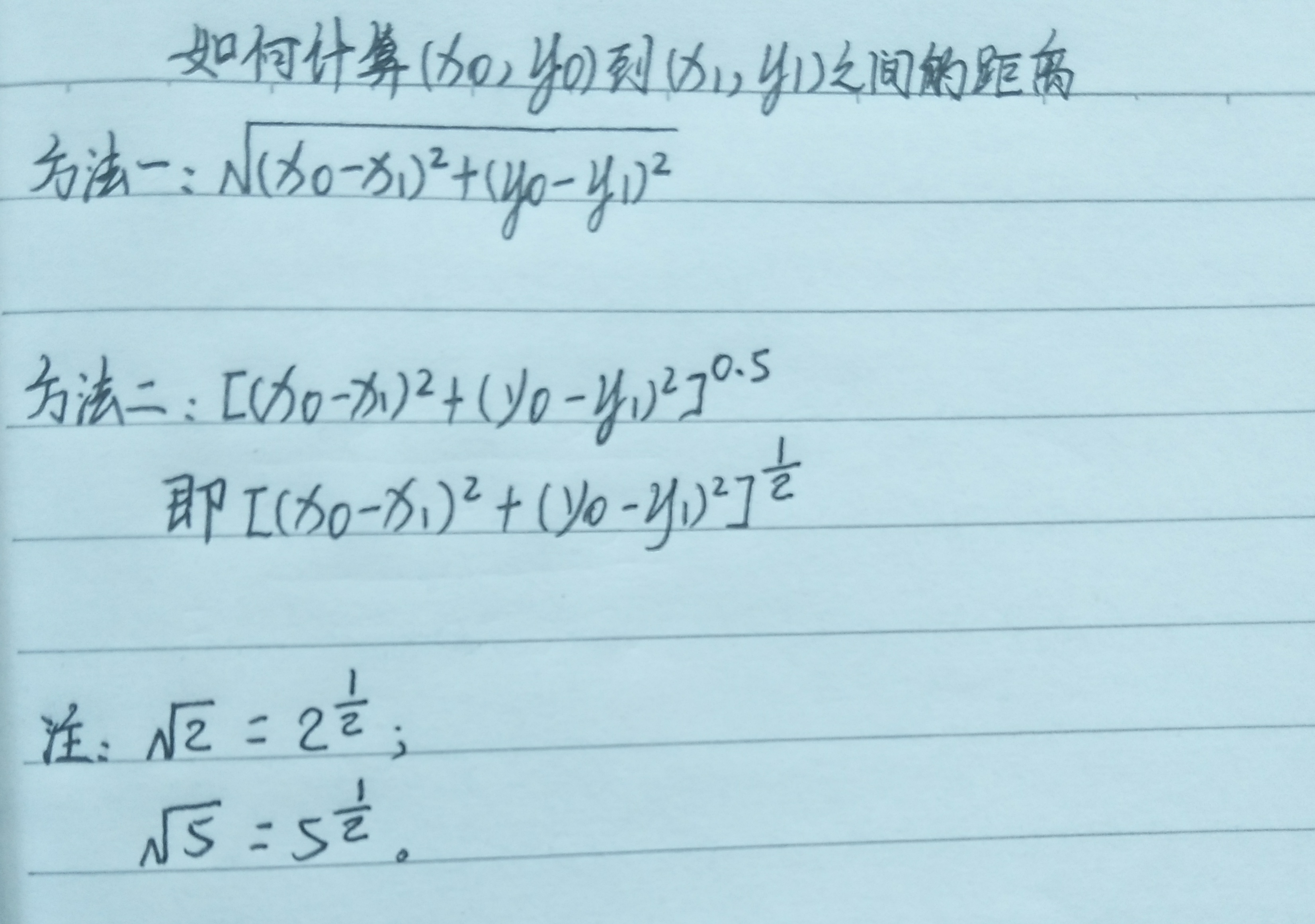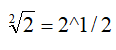• 今天小编就为大家分享一篇Python求两点之间的直线距离(2种实现方法)，具有很好的参考价值，希望对大家有所帮助。一起跟随小编过来看看吧
• 没有任何需要绘制或任何东西,只是比较,找到列表最接近的个.import math # 'math' needed for 'sqrt'# Distance functiondef distance(xi,xii,yi,yii):sq1 = (xi-xii)*(xi-xii)sq2 = (yi-yii)*(yi-yi...

我需要创建一个列表,找到最接近的两个点,然后打印出来.如何比较列表中的每个点？

没有任何需要绘制或任何东西,只是比较点,找到列表中最接近的两个.

import math # 'math' needed for 'sqrt'

# Distance function

def distance(xi,xii,yi,yii):

sq1 = (xi-xii)*(xi-xii)

sq2 = (yi-yii)*(yi-yii)

return math.sqrt(sq1 + sq2)

# Run through input and reorder in [(x, y), (x,y) ...] format

oInput = ["9.5 7.5", "10.2 19.1", "9.7 10.2"] # Original input list (entered by spacing the two points).

mInput = [] # Manipulated list

fList = [] # Final list

for o in oInput:

mInput = o.split()

x,y = float(mInput), float(mInput)

fList += [(x, y)] # outputs [(9.5, 7.5), (10.2, 19.1), (9.7, 10.2)]

展开全文• 求两点间距离 /***** 求两点间距离*****/ float getDistance(CvPoint pointO, CvPoint pointA) { float distance; distance = powf((pointO.x - pointA.x), 2) + powf((pointO.y - pointA.y), 2); distance = ...

求两点间距离

#***** 求两点间距离*****
def getDist_P2P(Point0,PointA):
distance=math.pow((Point0-PointA),2) + math.pow((Point0-PointA),2)
distance=math.sqrt(distance)
return distance

已知直线上两点求直线的一般式方程

常用的直线方程有一般式 点斜式 截距式 斜截式 两点式等等。除了一般式方程，它们要么不能支持所有情况下的直线（比如跟坐标轴垂直或者平行），要么不能支持所有情况下的点（比如x坐标相等，或者y坐标相等）。所以一般式方程在用计算机处理二维图形数据时特别有用。

已知直线上两点求直线的一般式方程

已知直线上的两点P1(X1,Y1) P2(X2,Y2)， P1 P2两点不重合。则直线的一般式方程AX+BY+C=0中，A B C分别等于：

A = Y2 - Y1

B = X1 - X2

C = X2*Y1 - X1*Y2

点到直线的距离:P到AB的距离

#***** 点到直线的距离:P到AB的距离*****
#P为线外一点，AB为线段两个端点
def getDist_P2L(PointP,Pointa,Pointb):
"""计算点到直线的距离
PointP：定点坐标
Pointa：直线a点坐标
Pointb：直线b点坐标
"""
#求直线方程
A=0
B=0
C=0
A=Pointa-Pointb
B=Pointb-Pointa
C=Pointa*Pointb-Pointa*Pointb
#代入点到直线距离公式
distance=0
distance=(A*PointP+B*PointP+C)/math.sqrt(A*A+B*B)

return distance

展开全文python
• 用Python如何计算两点间距离

万次阅读 多人点赞 2020-03-02 17:27:36
Python如何计算两点间距离？ 第一步，我们先来看一下两点间距离公式。 两点间距离公式： ？？？这是个啥？ 是不是方法一还能看懂，方法二却一脸懵逼？ 请仔细看注释， 也就是说，根号2还可以写成2的...

用Python如何计算两点间距离？

第一步，我们先来看一下两点间的距离公式。

两点间距离公式：？？？这是个啥？是不是方法一还能看懂，方法二却一脸懵逼？

请仔细看注释，也就是说，根号2还可以写成2的0.5(也就是1/2)次方

这个是高中学过的知识，不知道你有没有想起来，哈哈......

第二步，我们需要学习一下pow()这个函数

pow(a,b)是计算a的b次方的一个函数

例如：pow(2,3)为2的3次方，为8

第三步，split()函数

split()函数是将数据按指定格式分割开，默认为空格

例1：

a = "0 1 3 5"

print(a.slpit())

将a中的数据以空格的分割开，并返回一个['0', '1', '3', '5']形式的列表

例2：

a = 0,1,3,5

print(s.split(","))

将a中的数据以逗号分割开，并返回一个['0', '1', '3', '5']形式的列表

第四步 format()格式化

format()格式化与%s, %d, %f有异曲同工之效，都是先为数据占一个位，而后补充数据

例1：

weekday = "星期一"

print('今天是%s' % weekday)

显示为：今天是星期一

例2：

weekday = "星期一"

print('今天是{}'.format(weekday))

第五步，我们看一下具体的代码~

从键盘上输入4个数字，各数字采用空格分隔，对应变量为x0, y0, x1, y1。计算两点(x0, y0)和(x1, y1)之间的距离。在屏幕上输出这个距离，保留2位小数。例如，键盘输入0 1 3 5，屏幕输出5.00

#输入数据
ntxt=input('请输入4个数字(空格分隔):')
#利用split()函数，通过空格将数据分隔开并赋值给变量nls
nls=ntxt.split()
#利用eval()函数将字符串类型转化为数据类型；
#nls，将nls中的第零个数据赋值给变量x0
x0=eval(nls)
#nls，将nls中的第一个数据赋值给变量y0
y0=eval(nls)
#nls，将nls中的第二个数据赋值给变量x1
x1=eval(nls)
#nls，将nls中的第三个数据赋值给变量y1
y1=eval(nls)
#利用pow()函数计算两点间距离
r=pow(pow(x1-x0,2)+pow(y1-y0,2),0.5)
#打印数据；其中2f表示保留两位小数
print('{:.2f}'.format(r))

注：print('{:.2f}'.format(r))的意思是：用{:.2f}先占一个位置; .2f表示保留两位小数。

展开全文• 主要为大家详细介绍了python广度优先搜索得到两点间最短路径，具有一定的参考价值，感兴趣的小伙伴们可以参考一下
• python求两点之间的距离

千次阅读 2020-01-08 11:18:59
def cal_distance(p1, p2): return math.sqrt(math.pow((p2 - p1), 2) + math.pow((p2 - p1), 2)) 欢迎使用Markdown编辑器 你好！ 这是你第一次使用 Markdown编辑器 所展示的欢迎页。...
def cal_distance(p1, p2):
return math.sqrt(math.pow((p2 - p1), 2) + math.pow((p2 - p1), 2))

展开全文python
• 我按照以下方式实现了上述评论的讨论内容；计算是在您提取的二维灰色矩阵上进行的。我已经为输入到cluster的矩阵的感兴趣的值指定了0和1，在本例是KMeans，尽管可以根据需要用其他模型替换它。import cv2import ...
• 已知经纬度坐标求两点间距离，用python表示 已知地球上任意两点（lon1, lat1）,（ lon2, lat2）的经纬度坐标，求两点间距离用haversine表示： 1.首先先将经纬度坐标的角度化成弧度（mlon1,mlat1）,(mlon2,mlat2) 2...python
• 今天小编就为大家分享一篇python计算个地址之间的距离方法，具有很好的参考价值，希望对大家有所帮助。一起跟随小编过来看看吧
• 刚开始接触python的时候，有些内容，就感觉自己好像是在学习电脑端的数学知识，比如接下来经常会碰到的问题，使用python求两坐标距离，一起来看下吧~语法：#-*-coding:utf-8-*-importmath以下的类是得到一个class...
• 任意维度两点间距离计算任意维坐标中两点之间距离:import math def distance(p0,p1,digits=2): a=map(lambda x: (x-x...
• python编写，已知两点经纬度及高程坐标，求两点间距离的函数
• 计算出两点之间的距离日常旁白：本人是一枚生物学的学生，由于对生物信息学特别感兴趣，于是想自学生物信息学（新手莫怪）。了解到生物信息学要有编程基础，尤其是要会一门编程语言，例如：R语言、Python、Perl等，...
• 今天小编就为大家分享一篇python实现个经纬度之间的距离和方位角的方法，具有很好的参考价值，希望对大家有所帮助。一起跟随小编过来看看吧
• 方法一： #导入math包 import math #定义的函数 class Point: def __init__(self,x=0,y=0): self.x=x self.y=y def getx(self): return self.x def gety(self): return self.y ...
• 给定A(x1, y1), B(x2, y2)两点坐标，计算它们距离。 输入： 输入包含四个实数x1, y1, x2, y2，分别用空格隔开，含义如描述。其中0≤x1,x2,y1,y2≤100。 输出： 输出占一行，包含一个实数d，表示A, B...
• 欧式距离（L2范数）是最易于理解的一种距离计算方法，源自欧式空间中两点间距离公式（如下图所示）。   #导入math包 import math #定义点的函数 class Point: def __init__(self,x=0,y=0): self.x=x self.y...
• 在我的项目,我需要计算存储在数组的每个之间的欧几里德距离.入口数组是2D numpy数组,其中3列是坐标(x,y,z),每行定义一个新点.我通常在我的测试用例使用5000 – 6000.我的第一个算法使用Cython和我的第二个...
• #coding:UTF-8 """ Python implementation of Haversine formula Copyright (C) <2009> Bartek Górny This program is free software: you can redistribute it and/or modify it under the terms of the GNU ...
• 已知地球上任意两点（lng1，lat1），（lng2， lat2）的经纬度坐标，求两点间距离可以利用 haversine 公式： 首先先将经纬度坐标的角度化成弧度（rlng1，rlat1），（rlng2，rlat2) 利用如下公式： 其中 a ...python haversine geopy 两点经纬度计算距离
• 如下所示： distances = np.sqrt(np.sum(np.asarray(airportPosition - x_vals)**2, axis=1)) ... 您可能感兴趣的文章:python计算个地址之间的距离方法Python实现的计算马氏距离算法示例Python实现计
• 比如A点经纬度(110.0123, 23.32435)，B点经纬度(129.1344,25.5465)，AB两点之间的距离。我们可以用haversine()函数距离结果。Python版本的haversine()如下所示：from math import radians, cos, sin, asin, ...
• 太难找了，自己写个博客记录一下 hypot()方法返回的欧几里德范数 sqrt(xx + yy). ...len = hypot(x1-x2, y1-y2) #（x1, y1）与（x2, y2）的欧氏距离 参考： https://www.jb51.net/article/66227.htm ...
• 主要介绍了Python实现的计算马氏距离算法,简单说明了马氏距离算法原理,并结合实例形式分析了Python实现与使用马氏距离算法的相关操作技巧,需要的朋友可以参考下
• 这种文章本来网上一搜一大把，但有的计算...参考原文：LBS 球面距离公式 from math import sin, asin, cos, radians, fabs, sqrt EARTH_RADIUS=6371 # 地球平均半径，6371km def hav(theta): s = sin(theta /python lbs 地图...

python中求两点间距离python 订阅